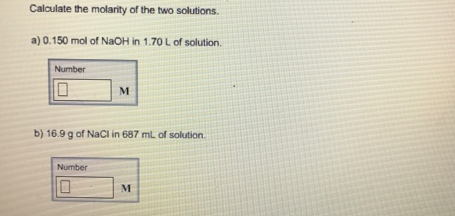Chemistry Molarity Solution: Calculate the molarity of the two solutions.a. 0.1...

🤓 Based on our data, we think this question is relevant for Professor Patterson's class at TXSTATE.

# Solution: Calculate the molarity of the two solutions.a. 0.150 mol of NaOH in 1.70 L of solution. b. 16.9 g of NaCl in 687 mL of solution.

Problem

Calculate the molarity of the two solutions.

a. 0.150 mol of NaOH in 1.70 L of solution.

b. 16.9 g of NaCl in 687 mL of solution.View Complete Written Solution